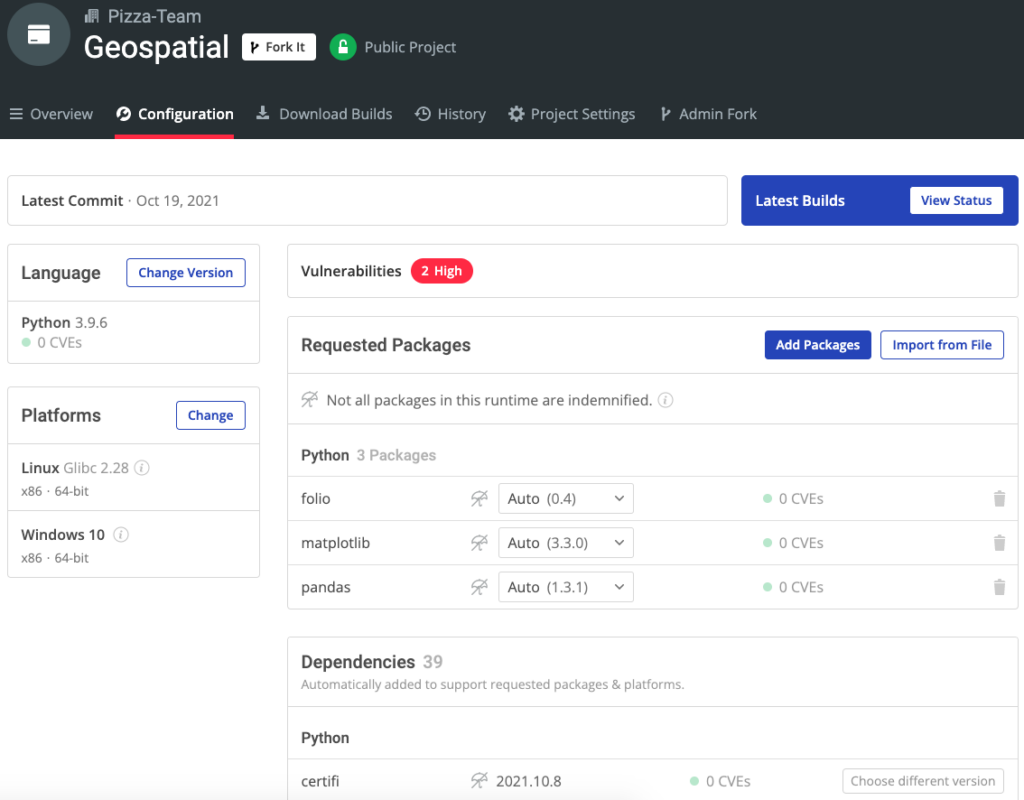# Human Impact on Amazon Rainforests: A Geospatial Analysis Using Python

The Amazonian rainforest used to be a global reserve of oxygen, but recent studies have shown that it has become a source of carbon instead. How did this happen? While most people acknowledge that humans were involved in the process, we can use Python and public datasets in order to better understand the impact of human actions on the Amazon.

In this article, we will use geospatial data to examine the static and dynamic pressures that the global economy puts on the Amazonian Basin. In order to work with geospatial data in Python, we’ll be using the following packages:

• GeoPandas: extends traditional Pandas to support spatial operations and geometric data types. It also allows you to plot vector maps.
• Folium: serves as a bridge between Python’s data wrangling capabilities and a Javascript library called Leaflet.js. It provides some handy methods for creating maps with interactive features.

There are two types of data for geospatial analysis:

• Vector Data: vectors refer to data structures that represent specific features on the Earth’s surface as well as attributes assigned to those features.
• Raster Data: raster data is represented by matrices of values which are rendered as pixels on a map. Each pixel value represents an area on the Earth’s surface.

We will only be working with vector data in this article, which will walk you through all the code needed to visualize the data over time, including:

1. Sourcing Geospatial Data
2. Plotting Geospatial Data with GeoPandas
3. Applying Mapping Context with Folium
4. Building a Timelapse of Fires

You can find the source for all the code in this article in my GitHub repository. xf

## Before You Start: Install The Geospatial Python Environment

To follow along with the code in this article, you can download and install our pre-built Geospatial environment, which contains a version of Python 3.9 and the packages used in this post, along with already resolved dependencies!

Or you could also use our State tool to install this runtime environment.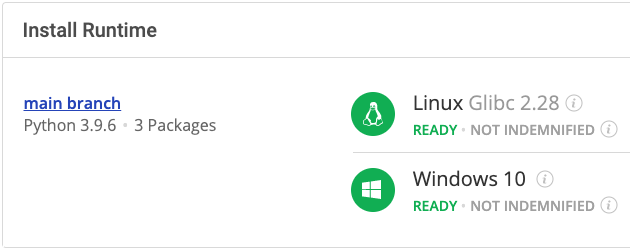For Windows users, run the following at a CMD prompt to automatically download and install our CLI, the State Tool along with the Geospatial runtime into a virtual environment:

`powershell -Command "& \$([scriptblock]::Create((New-Object Net.WebClient).DownloadString('https://platform.activestate.com/dl/cli/install.ps1'))) -activate-default Pizza-Team/Geospatial"`

For Linux users, run the following to automatically download and install our CLI, the State Tool along with the Geospatial runtime into a virtual environment:

`sh <(curl -q https://platform.activestate.com/dl/cli/install.sh) --activate-default Pizza-Team/Geospatial`

## 1–Sourcing Geospatial Data

There are many sources online where you can obtain geospatial data. The Amazon Geo-Referenced Socio-Environmental Information Network (RAISG) is a civil consortium of Amazonian countries (backed by international partners) that provides statistical data, geographic information, and different analyses of the Amazonian region. In this article, we’ll source our information from the following shapefiles located in RAISG’s catalog:

• RAISG limits: the perimeter of the Amazon Basin.
• Hydroelectric plants: shows the locations of hydroelectric plants that are either currently running, under construction, or planned.
• Oil: shows areas of oil exploration/exploitation.
• Mining blocks: shows areas of legal mining concessions.
• Illegal mining: shows areas of illegal mining.

## 2–Plotting Geospatial Data with GeoPandas

GeoPandas extends the Pandas package to add some extra capabilities to the classic Pandas DataFrames routine. You’ll need to import some packages and read the shapefiles that you’ve downloaded before you load the data:

```import os
import folium
import itertools
import pandas_alive
import pandas as pd
import geopandas as gpd
import matplotlib.pyplot as plt
from branca.element import Figure
from matplotlib.patches import Patch
from datetime import datetime, date, timedelta
from collections import defaultdict, OrderedDict
from folium.plugins import FastMarkerCluster, HeatMapWithTime
%matplotlib inline

As you can see in the image above, each file is loaded into a GeoDataFrame. GeoPandas supports several different file types, including ArcGIS shapefiles and GeoJSON. You can use the GeoDataFrame plot() function directly with some custom legends to get a glimpse of the maps since they all share the same Coordinate Reference System (CRS). Otherwise, you’ll need to reproject the data in order to get the correct mapping between the coordinates of the data and the places on the Earth. For example, try the following code:

```fig, ax = plt.subplots(figsize=(15,15))
legend_elements = [Patch(facecolor='green', label='Basin')
, Patch(facecolor='blue', label='Hydroelectric')
, Patch(facecolor='orange', label='Mining')
, Patch(facecolor='red', label='Ilegal mining')
, Patch(facecolor='black', label='Oil')
limits.plot(ax=ax, color='green',legend=True, label='AAAAA')
hydro.plot(ax=ax, color='blue')
mining.plot(ax=ax, color='orange')
ilegal_mining.plot(ax=ax, color='red')
oil.plot(ax=ax, color='black')
rails.plot(ax=ax, color='brown')
ax.legend(handles=legend_elements)
plt.show()
```

The above code example will generate the following map: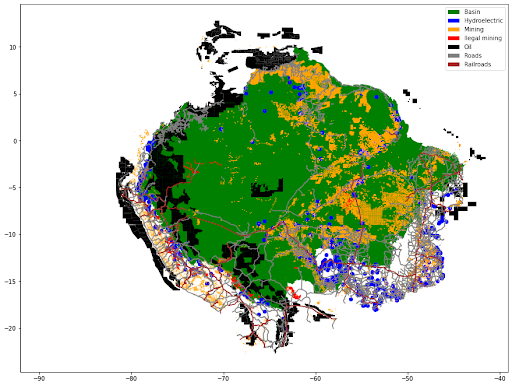At this point, the map lacks context. It’s just a blank space with some layers that statistically represent the data. To get more information, you can perform a deeper exploratory data analysis on each GeoDataFrame. For example, the hydroelectric DataFrame contains some interesting information: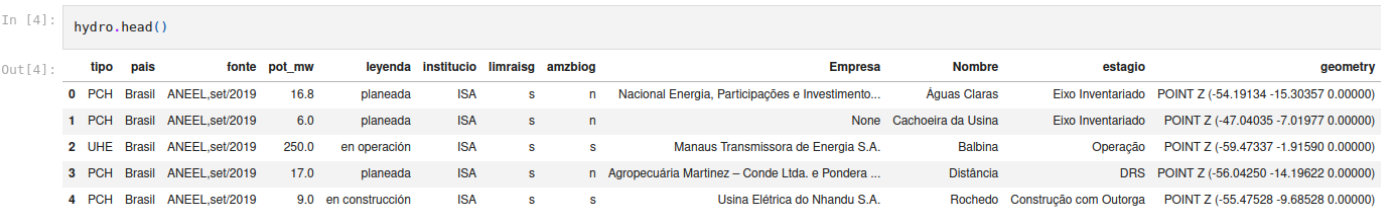Here, you can see future hydroelectric plants, the companies that are building them, and the amount of power that they will produce (in megawatts). Now, let’s add some context to the map as well as some interactive features.

## 3–Applying Mapping Context with Folium

There are many different ways to add a global or local map to contextualize your data. One common option is Cartopy, but Folium gives you two important features from the Leaflet.js library: interactivity and clusterization.

To provide regional context for the countries of the Amazon Basin, we’ll create a Folium map based around the centroid of the basin with the Terrain tiles like this:

```def hydro_markers( r, dest ):
color = 'lightblue'
if 'en constru' in r['leyenda']:
color = 'blue'
elif 'en opera' in r['leyenda']:
color = 'darkblue'
marker = folium.Marker(location=[r.geometry.y, r.geometry.x], popup='<b>{}</b><br> Country: {}, Status: {}'.format(r['Nombre'], r['pais'], r['leyenda']), icon=folium.Icon(color=color))
return None
fig = Figure(width=720,height=540)
limits_prj = limits.to_crs(epsg=3857)
limits['centroid'] = (limits_prj.centroid).to_crs(epsg=4326)
#creates a zmap centered on the abamzonian basin
m = folium.Map(location=(limits['centroid'].y,limits['centroid'].x), zoom_start=4, tiles = "Stamen Terrain", control_scale=True, prefer_canvas=True)
#adds the amazonian basin limits over the map
sim_geo = gpd.GeoSeries(limits.boundary).simplify(tolerance=0.001)
hydro.apply(lambda x: hydro_markers(x,m), axis=1)

There are other options we could have used, including a Stamen Toner and OpenStreetMap, but the Folium map should serve for our purposes.

The above code:

1. Simplifies the limits for the GeoDataFrame boundary
2. Adds it as a layer on the map
3. Places markers for each hydroelectric plant with the defined hydro_markers (we set the color of the markers based on the status of each row, then add them to the main map with descriptive popups. Note that Folium popups support HTML).

The result is a map with zoom capabilities and a marker for each hydroelectric plant that looks like this: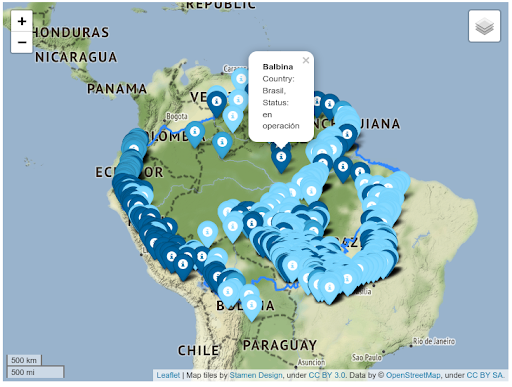As you can see, this map is far too crowded to be useful. To fix it, you can utilize Folium’s clustering capabilities. This code is similar to the code above, but it declares a FastMarkerCluster instance as a new layer on the map where each marker is located:

```fig = Figure(width=720,height=540)
m = folium.Map(location=(limits['centroid'].y,limits['centroid'].x), zoom_start=4, tiles = "Stamen Terrain", control_scale=True, prefer_canvas=True)
#adds the amazonian basin limits over the map
sim_geo = gpd.GeoSeries(limits.boundary).simplify(tolerance=0.001)
hydro_cluster = FastMarkerCluster(data=[], name="Hidroelectrics")
hydro.apply(lambda x: hydro_markers(x,hydro_cluster), axis=1)

The resulting map clusters the markers in regions. You can click the cluster to zoom in on the region and see each individual marker: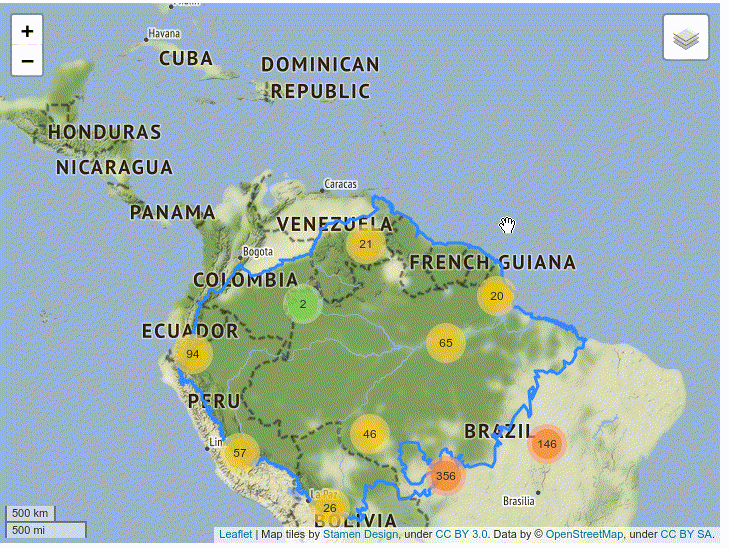## 4–Building a Timelapse of Fires

The previous maps were built with static data, but you can also use time series datasets that can give us a better idea of how the Amazon is changing over time. The Global Fire Emissions Database provides a shapefile (which is updated daily) of the fires detected in the Amazonian rainforest through the Visible Infrared Imaging Radiometer Suite. Each datapoint contains the type of fire and the starting and ending date (DOY), among other characteristics: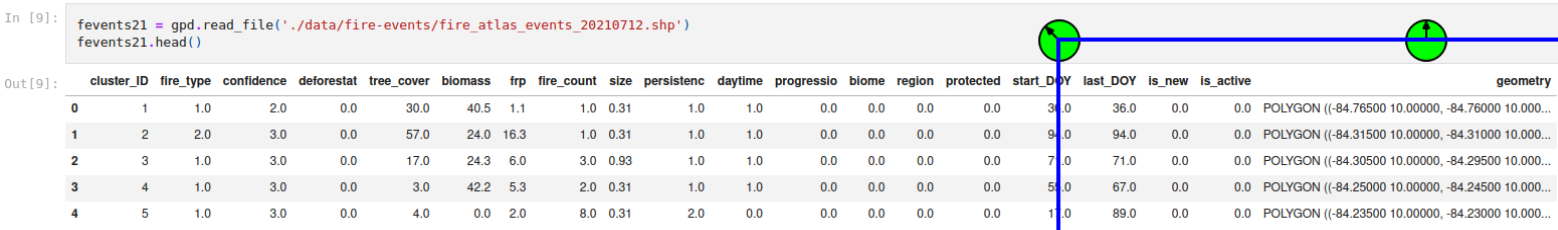As you can see, the geometry is a polygon.  We can construct a DataFrame that transforms the start_DOY and last_DOY columns into a Python date, and then use the polygon centroid to build a Folium HeatMapWithTime that will render the points as a dynamic heat map:

```#only fires affecting the RASIG biome defined boundary are considered
fires_2021 = fevents21.loc[fevents21['biome'] == 1][['geometry','start_DOY','last_DOY','fire_type','size']]
days_map = {}
#Populates a map with a key per day with a list of the centroids of the fires that are active
for day in fires_2021['start_DOY'].sort_values().unique():
df = fires_2021.loc[fires_2021['start_DOY'] == day]
for _,r in df.iterrows():
for d in range(0,int(r['last_DOY'] - r['start_DOY'])+1):
k = (date(2021, 1, 1) + timedelta(days=(int(day+d) - 1)))
if k not in days_map:
days_map[k] = []
days_map[k].append( [r['geometry'].centroid.y, r['geometry'].centroid.x] )
days_map = OrderedDict(sorted(days_map.items(), key=lambda t: t))
fig = Figure(width=720,height=540)
m = folium.Map(location=(limits['centroid'].y,limits['centroid'].x), zoom_start=4, tiles = "Stamen Terrain", control_scale=True, prefer_canvas=True)
#adds the amazonian basin limits over the map
sim_geo = gpd.GeoSeries(limits.boundary).simplify(tolerance=0.001)
#Creates a layer with the time series of fires, the index is used to render the current day of the animation
hm = HeatMapWithTime(data=list(days_map.values()),
index=[d.strftime("%d-%m-%Y") for d in days_map.keys()],
name='Fires 2021',
auto_play=True,
max_opacity=0.3,
min_speed=10,
max_speed=24,
speed_step=1)

The resulting map displays the day (analyzed as a label), and clusters the data points using a heat colormap. It also contains controls for the speed and frame of the animation.

As you can see, the map shows that the southeastern region of Amazonia has a huge amount of fires, which is consistent with conclusions of formal studies.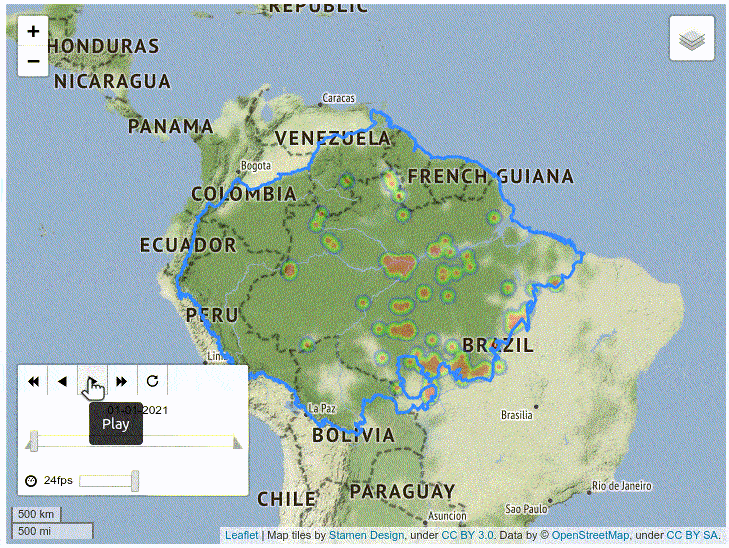Finally, plotting the time series data of the fire types reveals their purpose or origin:

• Type 1 (blue line) are savanna and grassland fires
• Type 2 (orange line) are for small clearings and agriculture
• Type 3 (green line) are understory
• Type 4 (red line) are deforestation fires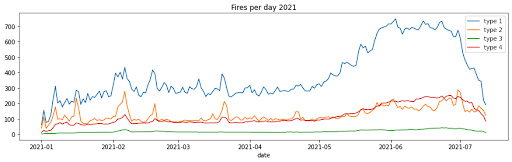Clearing the rainforest for cattle rearing creates more areas of savanna and grassland for the cattle to graze on, but as the chart above shows, these same grasslands are extremely susceptible to wildfires.

## Conclusions – Geospatial Data Analysis Using Python

The phenomenon of global climate change shows that while humanity continues to deny our impact on the ecosystem, we can’t hide from the consequences. Using Python’s GIS tools can help you make up your own mind about human impact on climate change.

For further study, you might want to see what a precipitation map of the region looks like over time. More fires mean fewer trees, which in turn mean less rain, thus making the dry season even more susceptible to uncontrolled fires. This kind of negative feedback loop is at the heart of how the Amazon rainforest has recently shifted from a CO2 sink to a C02 producer, and represents a dramatic turning point in the fight to limit the growth of greenhouse gases.

In this article, we used public datasets to give you a glimpse into the power of geographical data analysis. You can learn more:

Next steps: